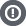Hello Guest

• 0 Replies
• 5220 Views####dangerdoc

•• 75
• Thats right... Nikola Tesla`value += fractX       * fractY       * fractZ       * noise[x1][y1][z1];   value += fractX       * (1 - fractY) * fractZ       * noise[x1][y2][z1];   value += (1 - fractX) * fractY       * fractZ       * noise[x2][y1][z1];   value += (1 - fractX) * (1 - fractY) * fractZ       * noise[x2][y2][z1];   value += fractX       * fractY       * (1 - fractZ) * noise[x1][y1][z2];   value += fractX       * (1 - fractY) * (1 - fractZ) * noise[x1][y2][z2];   value += (1 - fractX) * fractY       * (1 - fractZ) * noise[x2][y1][z2];   value += (1 - fractX) * (1 - fractY) * (1 - fractZ) * noise[x2][y2][z2];`means. I cant make sense of code like that without an explanation. How do you process the information, and what is the reason for each line of code? If I learn to do 3-D trillinear interpolation, I am willing to make a tutorial that explains it to others like me. Thank you for reading this post, and thank you even more if you are willing to walk me through this.